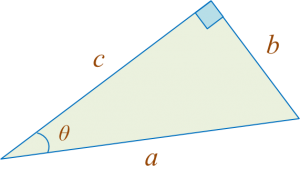# PT3 Mathematics 2016, Question 5

Question 5 (a):
Diagram 5.1 shows a right-angled triangle.Match cos θ and tan θ to the correct trigonometric ratio.Solution
:Question 5 (b):
Diagram 5.2 shows a trapezium ABCD.Calculate the area, in cm2, of the shaded region.

Solution:

Question 5 (c):
(i) Simplify a-2 ÷ a3
(ii) It is given that 53x + 4 ÷ 53 = 125.
Find the value of x.

Solution:
(i)
a-2 ÷ a3 = a-2-3
= a-5

(ii)
53x + 4 ÷ 53 = 125
53x + 4 – 3 = 53
53x + 1 = 53
3x + 1 = 3
3x = 2
x =